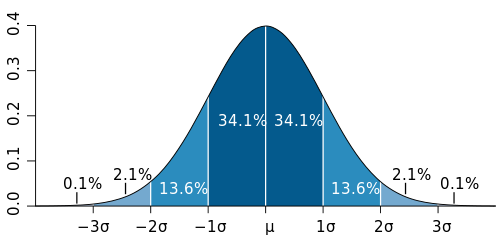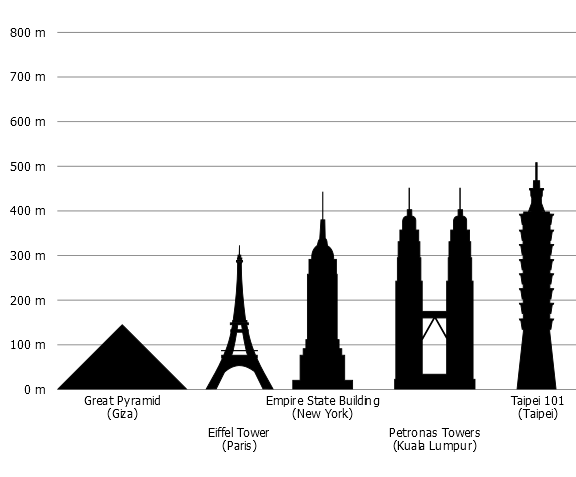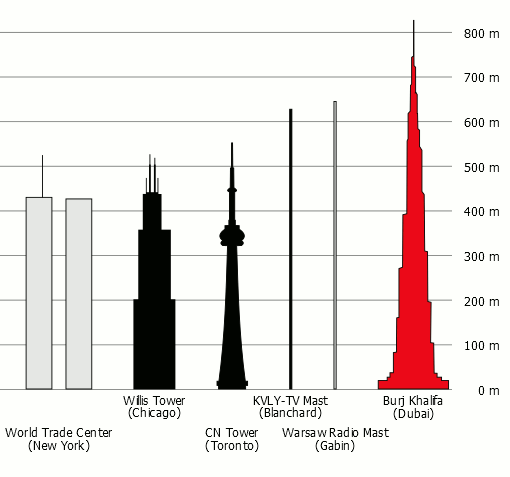# Standard Deviation

The Variance and the Standard Deviation are measures of spread of the data indicating how far the data is away from the normal (mean).

When data such as people's heights, weights, intelligence, school test marks and so forth are graphed, they tend to create a "bell-shaped curve" or normal distribution curve.

Mathematical Symbols:

• Mean μ
• Variance σ 2
• Standard Deviation σ

Normal Distribution Graph:A Normal Distribution curve with sufficient data has the following features:

• The Mean is in the middle.
• The Highest Score is the Mean plus 3 Standard Deviations.
• The Lowest Score is the Mean minus 3 Standard Deviations.

## Finding the Standard Deviation of a Population

• Calculate the Mean of all the data (by adding up all the numbers and dividing by how many numbers there are).
• For each data number, subtract it from the Mean.
• Square this difference. This is called the Squared Difference.
• Find the Mean of the Squared Differences. This is called the Variance.
• Find the Square Root of the Variance. This is called the Standard Deviation.

## Example One - Intelligence Quotient (IQ)In most IQ tests, the "average" or Mean IQ is about 100 and the Standard Deviation is about 15. The vast majority of people have IQs between 70 and 130. However, famous physicists Albert Einstein and Stephen Hawking (in the photographs above) are thought to have IQs of about 145 (which is equal to the Mean plus 3 Standard Deviations). Refer to the instructions above to calculate the mean, the variance and the standard deviation of the IQ data of the following fictitious people listed in this table.

 PERSON IQ Al 76 Bert 80 Cuc 89 David 96 Eng 100 Fran 102 Gianni 106 Helena 108 Ines 122 Jack 130

Answer:

 PERSON IQ IQ DIFFERENCE FROM THE MEAN SQUARE OF THE IQ DIFFERENCE FROM THE MEAN Al 76 – 24.9 620.01 Bert 80 – 20.9 436.81 Cuc 89 – 11.9 141.61 David 96 – 4.9 24.01 Eng 100 – 0.9 0.81 Fran 102 + 1.1 1.21 Gianni 106 + 5.1 26.01 Helena 108 + 7.1 50.41 Ines 122 + 21.1 445.21 Jack 130 + 29.1 846.81 MEAN IQ = 100.9 MEAN OF SQUARED DIFFERENCES = 259.29

Mean = 100.9
Variance = 259.29

 Standard Deviation = square root of variance = √ 259.29 = 16.1

## Example Two - World's Tallest Structures

 STRUCTURE HEIGHT IN METRES Great Pyramid of Giza 139 Eiffel Tower 300 Empire State 381 Petronas Towers 452 Taipei 101 509 World Trade Centre 526 CN Tower 553 KVLY-TV Mast 629 Warsaw Radio Mast 646 Burj Khalifa 830The graph and the table show the heights of ten of the world's tallest structures. Draw a table and then calculate the:
(a) mean
(b) variance
(c) standard deviation.

Answer:

 STRUCTURE HEIGHT IN METRES HEIGHT DIFFERENCE FROM THE MEAN SQUARE OF THE HEIGHT DIFFERENCE FROM THE MEAN Great Pyramid of Giza 139 – 357.5 127 806.25 Eiffel Tower 300 – 196.5 38 612.25 Empire State 381 – 115.5 13 340.25 Petronas Towers 452 – 44.5 1 980.25 Taipei 101 509 + 12.5 156.25 World Trade Centre 526 + 29.5 870.25 CN Tower 553 + 56.5 3 192.25 KVLY-TV Mast 629 + 132.5 17 556.25 Warsaw Radio Mast 646 + 149.5 22 350.25 Burj Khalifa 830 + 333.5 111 222.25 MEAN HEIGHT = 496.5 m MEAN OF SQUARED DIFFERENCES = 22 597.525

Mean = 496.5 m
Variance = 22 597.525

 Standard Deviation = square root of variance = √ 22 597.525 = 150.32 m

## Questions to Consider

Which building is closest to the "average" height of these ten structures?
Why are some of the differences from the mean positive and others negative?
Why are these differences squared?

## Maths Fun

Find out how to get the Mean and the Standard Deviation on your calculator without using a table. The symbols are:

• Mean x
• Standard Deviation σn-1